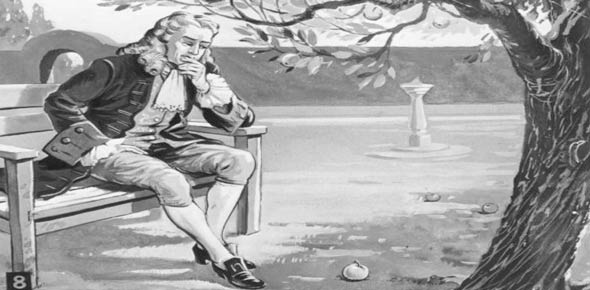# Quiz: Laws On Motion

10 Questions | Total Attempts: 45Settings.

Related Topics
• 1.
Which situation shows unbalanced forces?
• A.

A

• B.

B

• C.

C

• D.

D

• E.

E

• F.

A and C

• G.

C and D

• 2.
A book has a mass of 850 grams. What is its mass in kg?
• A.

0.85

• B.

8.5

• C.

85

• D.

850000

• 3.
A piece of rock has a mass of 2500 grams. What is its weight in Newtons? (Type numbers only)
• 4.
The mass of a bag is 750 grams. If gravity on Planet Z is 1/4 that of the Earth, what is the mass of the bag on Planet Z?
• A.

7.5 kg

• B.

7.5 N

• C.

750 g

• D.

750 N

• 5.
Which of the following cars will accelerate the farthest?
• A.

A

• B.

B

• C.

C

• D.

D

• 6.
______________________ is the amount of matter in an object. (Type small letters only)
• 7.
_________________ is the force that opposes motion. (Type small letters only)
• 8.
Which law of motion states that: "For every action, there is an equal and opposite reaction?
• A.

1st law

• B.

2nd law

• C.

3rd law

• D.

4th law

• 9.
What type of friction is shown by the image? (Type only small letters)
• 10.
The mass of a box is 250 grams. What is its weight in Newtons on Earth? (Type numbers only).